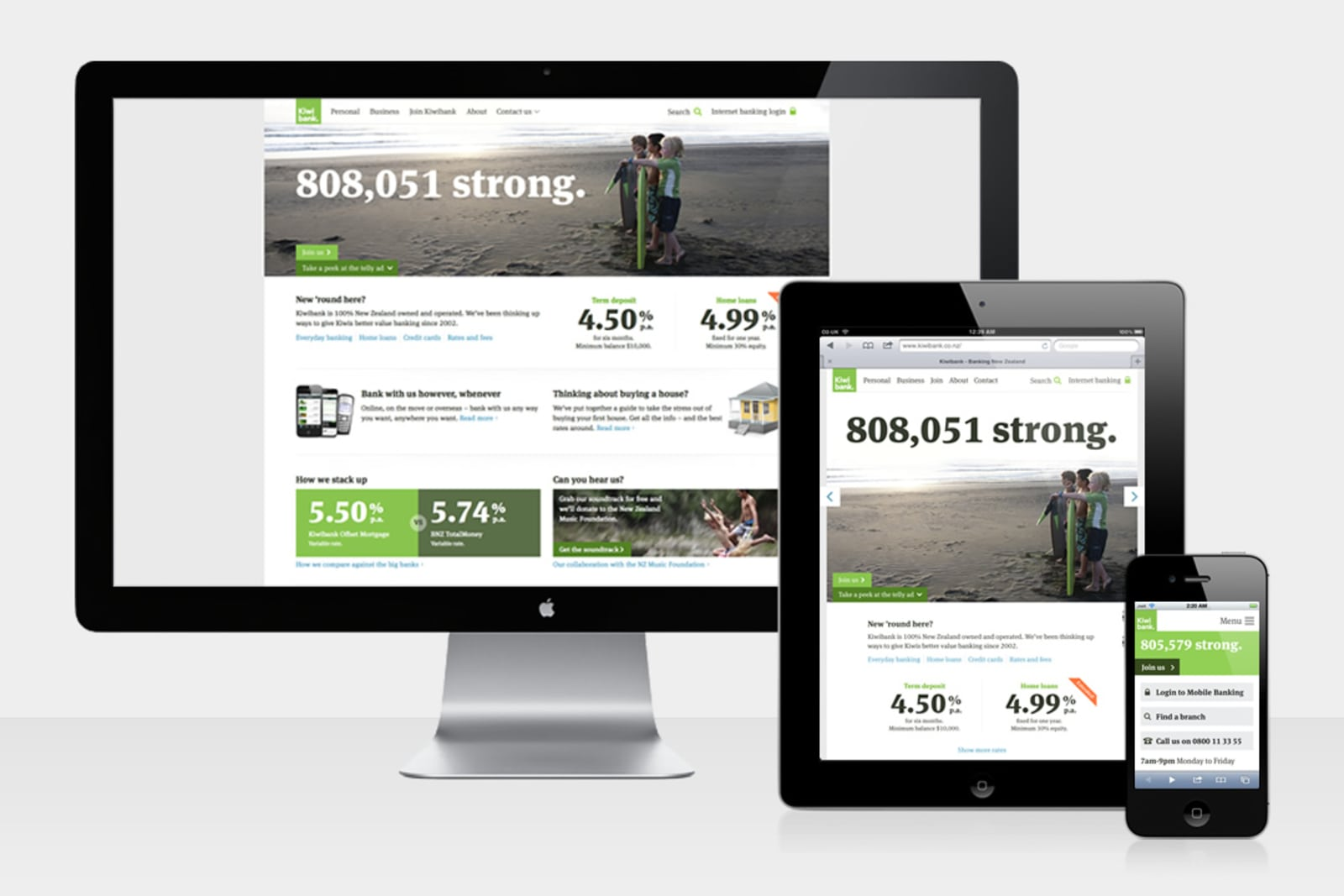Top

# Kiwibank – responsive homepageself.__wrap_b=(t,n,e)=>{e=e||document.querySelector(`[data-br="\${t}"]`);let s=e.parentElement,r=R=>e.style.maxWidth=R+"px";e.style.maxWidth="";let o=s.clientWidth,i=s.clientHeight,c=o/2-.25,l=o+.5,u;if(o){for(;c+1<l;)u=Math.round((c+l)/2),r(u),s.clientHeight===i?l=u:c=u;r(l*n+o*(1-n))}e.__wrap_o||(e.__wrap_o=new ResizeObserver(()=>{self.__wrap_b(0,+e.dataset.brr,e)})).observe(s)};self.__wrap_b(":R1l6:",1)

As far as we can tell, this was one of the first responsive bank homepages in the world.